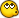# calculating battery capacity

#### butcherman

Moderator
How does one calculate how long a battery will supply power for.

example: pump rating 18v 26w battery 74Ah.

is it W/V=A and the simply divide the Ah by the A?

If so the answer to this problem is 51.39 hours?

Can this be right?

#### tjiPPi

Your equation is correct (P=VI), but the calculation is not necessarily as straight forward.

You'll need to give more details:

1) I'm assuming the 72Ah battery is 12V, is this correct?

2) Do you have anything connected between the battery and pump or are you running the 18V pump at 12V (if battery is 12V)?

Things that normally influence the calculation is:
- Converter efficiency (things like inverters, buck -, boost converters, regulators)
- Maximum discharge of battery before damage. (For lead acid battery I think it is between 50% and 70% - don't quote me on this)
- Discharge rating of the battery (exceeding this will mean that the Ah rating of the battery is not a true representation any more.)

#### butcherman

Moderator
Your equation is correct (P=VI), but the calculation is not necessarily as straight forward.

You'll need to give more details:

1) I'm assuming the 72Ah battery is 12V, is this correct?

2) Do you have anything connected between the battery and pump or are you running the 18V pump at 12V (if battery is 12V)?

Things that normally influence the calculation is:
- Converter efficiency (things like inverters, buck -, boost converters, regulators)
- Maximum discharge of battery before damage. (For lead acid battery I think it is between 50% and 70% - don't quote me on this)
- Discharge rating of the battery (exceeding this will mean that the Ah rating of the battery is not a true representation any more.)
Its a Tunze pump 1073.05 the power supply it normally uses is 220v converted to 24v but the pump can be run on anything from 10v to 28v.

Yes the Battery is 12v 74Ah
so on back up power it runs straight off 12v The pump has a safety connector that automaticallty switches power sources the pump does not even flinch when it switches.

the only ratings i can find for the diffrent modes are
15v 20w
18v 26w
24v 43w

I dont know what the 12v watts ratting is but i suspect its lower than 1.33A

i run the thing for 12 hours and turned the power back on cause i went to bed and did not want it running on battery power unattended.

#### tjiPPi

Yes, for 12V the power consumption should be less.

Lets work on the assumption that the switch over circuit is a simple relay type with no losses and we are within the battery discharge rate. Also, lets use the current rating at 15V (it should be less than this so we take this as worst case).
Thus: using P=V*I (as you correctly did)
I= 1.33A @15V

74Ah/1.33A = 55.5h

But this is the point where the battery is 100% discharged. Doing this to a lead acid battery kills it. Normally for lead acid you dont want to discharge it more than 50%.

This means 27h in this case.
The load (1.33A) should be within the discharge rate. If it's not, the 74Ah figure does not apply 100% anymore. Just double check the discharge rate on the battery (normally an hour rate like: 74Ah @ xx hours).
74/xx hours > 1.33A for above to be relatively accurate.

Last edited:

#### butcherman

Moderator
The load (1.33A) should be within the discharge rate. If it's not, the 74Ah figure does not apply 100% anymore. Just double check the discharge rate on the battery (normally an hour rate like: 74Ah @ xx hours). 74/xx hours > 1.33A for above to be relatively accurate.
Wow thats fot your help. this is complicated this particular battery says its realed for 20 hours discharge i think. and is 74Ah.
So dose this mean i can safely run the pump 20 hours?

#### tjiPPi

Pleasure!
The short answer is: Yes.

The 20h discharge rate means that the battery will supply 3.7A continuous for 20 hours. (74Ah/20h).
Thus, in your case you will be able to run the pumps for 55h (battery will be 100% discharged though).

For interest:
If you were to pull more than 3.7A out of the battery, you cannot rely on the 74Ah spec any more. Most of the time there is a discharge rate graph on the datasheet of the battery (can be found on manufacturer website) that you use to calculate a new battery capacity that relates to the load connected to the battery. For example if you pull 4A you cannot say 74Ah/4=18.5h, but rather 65Ah(you get this from the graph)/4=16.25h. (btw i used made-up values in example)

Hope this helps

Last edited:

#### butcherman

Moderator
Thanks for your help. this system is for my expected load sheadding so it has to last potentially 3.5hours everyday accourding to the schedule so i think this will work very well.